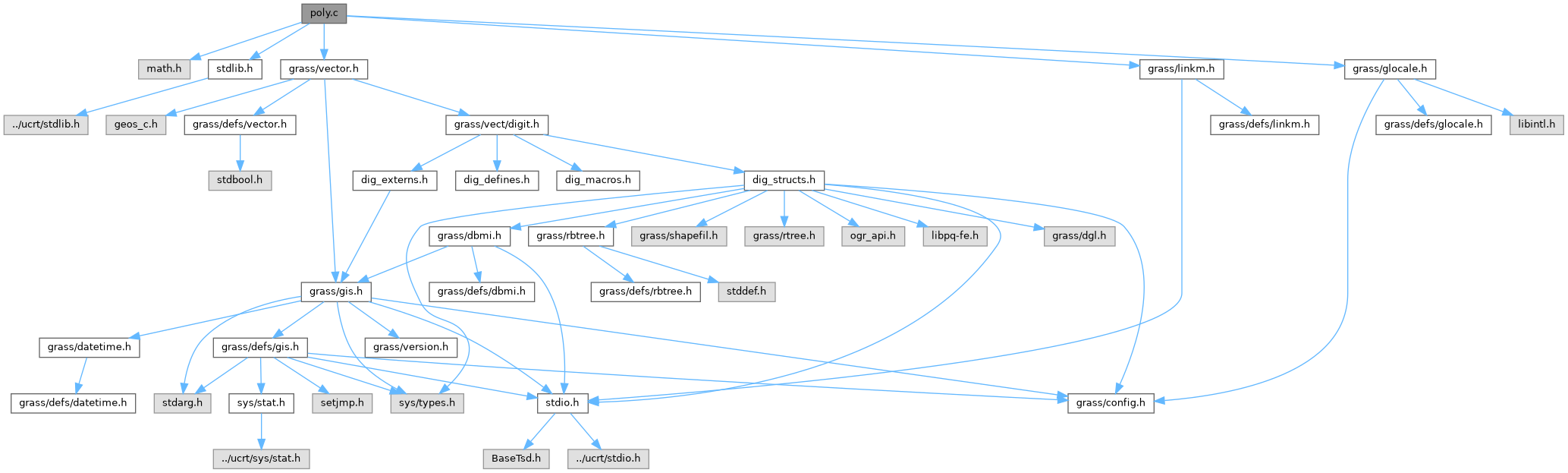GRASS GIS 8 Programmer's Manual  8.2.2dev(2023)-5c00b4e944
Vlib/poly.c File Reference

Vector library - polygon related fns. More...

`#include <math.h>`
`#include <stdlib.h>`
`#include <grass/vector.h>`
`#include <grass/linkm.h>`
`#include <grass/glocale.h>`
Include dependency graph for Vlib/poly.c:Go to the source code of this file.

## Functions

int Vect__intersect_y_line_with_poly ()

int Vect__intersect_x_line_with_poly ()

int Vect_get_point_in_area (const struct Map_info *Map, int area, double *X, double *Y)
Get point inside area and outside all islands. More...

int Vect__intersect_y_line_with_poly (const struct line_pnts *Points, double y, struct line_pnts *Inter)

int Vect__intersect_x_line_with_poly (const struct line_pnts *Points, double x, struct line_pnts *Inter)

int Vect_get_point_in_poly (const struct line_pnts *Points, double *X, double *Y)
Get point inside polygon. More...

int Vect_find_poly_centroid (const struct line_pnts *points, double *cent_x, double *cent_y)
Get centroid of polygon. More...

int Vect_get_point_in_poly_isl (const struct line_pnts *Points, const struct line_pnts **IPoints, int n_isles, double *att_x, double *att_y)
Get point inside polygon but outside the islands specifiled in IPoints. More...

int Vect_point_in_poly (double X, double Y, const struct line_pnts *Points)
Determines if a point (X,Y) is inside a polygon. More...

int Vect_point_in_area_outer_ring (double X, double Y, const struct Map_info *Map, int area, struct bound_box *box)
Determines if a point (X,Y) is inside an area outer ring. Islands are not considered. More...

int Vect_point_in_island (double X, double Y, const struct Map_info *Map, int isle, struct bound_box *box)
Determines if a point (X,Y) is inside an island. More...

## Detailed Description

Vector library - polygon related fns.

Higher level functions for reading/writing/manipulating vectors.

(C) 2001-2009 by the GRASS Development Team

This program is free software under the GNU General Public License (>=v2). Read the file COPYING that comes with GRASS for details.

Definition in file Vlib/poly.c.

## ◆ Vect__intersect_x_line_with_poly() [1/2]

 int Vect__intersect_x_line_with_poly ( )

## ◆ Vect__intersect_x_line_with_poly() [2/2]

 int Vect__intersect_x_line_with_poly ( const struct line_pnts * Points, double x, struct line_pnts * Inter )

Definition at line 172 of file Vlib/poly.c.

## ◆ Vect__intersect_y_line_with_poly() [1/2]

 int Vect__intersect_y_line_with_poly ( )

## ◆ Vect__intersect_y_line_with_poly() [2/2]

 int Vect__intersect_y_line_with_poly ( const struct line_pnts * Points, double y, struct line_pnts * Inter )

Definition at line 128 of file Vlib/poly.c.

## ◆ Vect_find_poly_centroid()

 int Vect_find_poly_centroid ( const struct line_pnts * points, double * cent_x, double * cent_y )

Get centroid of polygon.

Parameters
 points polygon [out] cent_x,cent_y centroid coordinates
Returns
0 on success
-1 on error

Definition at line 363 of file Vlib/poly.c.

References line_pnts::n_points, line_pnts::x, and line_pnts::y.

## ◆ Vect_get_point_in_area()

 int Vect_get_point_in_area ( const struct Map_info * Map, int area, double * X, double * Y )

Get point inside area and outside all islands.

Take a line and intersect it with the polygon and any islands. sort the list of X values from these intersections. This will be a list of segments alternating IN/OUT/IN/OUT of the polygon. Pick the largest IN segment and take the midpoint.

Parameters
 Map vector map area area id [out] X,Y point coordinateds
Returns
0 on success
-1 on error

Definition at line 56 of file Vlib/poly.c.

## ◆ Vect_get_point_in_poly()

 int Vect_get_point_in_poly ( const struct line_pnts * Points, double * X, double * Y )

Get point inside polygon.

This does NOT consider ISLANDS!

Parameters
 Points polygon [out] X,Y point coordinates
Returns
0 on success
-1 on error

Definition at line 211 of file Vlib/poly.c.

## ◆ Vect_get_point_in_poly_isl()

 int Vect_get_point_in_poly_isl ( const struct line_pnts * Points, const struct line_pnts ** IPoints, int n_isles, double * att_x, double * att_y )

Get point inside polygon but outside the islands specifiled in IPoints.

Take a line and intersect it with the polygon and any islands. sort the list of X values from these intersections. This will be a list of segments alternating IN/OUT/IN/OUT of the polygon. Pick the largest IN segment and take the midpoint.

Parameters
 Points polygon (boundary) IPoints isles (list of isle boundaries) n_isles number of isles [out] att_x,att_y point coordinates
Returns
0 on success
-1 on error

Definition at line 457 of file Vlib/poly.c.

## ◆ Vect_point_in_area_outer_ring()

 int Vect_point_in_area_outer_ring ( double X, double Y, const struct Map_info * Map, int area, struct bound_box * box )

Determines if a point (X,Y) is inside an area outer ring. Islands are not considered.

Parameters
 X,Y point coordinates Map vector map area area id box area bounding box
Returns
0 - outside
1 - inside
2 - on the boundary

Definition at line 856 of file Vlib/poly.c.

## ◆ Vect_point_in_island()

 int Vect_point_in_island ( double X, double Y, const struct Map_info * Map, int isle, struct bound_box * box )

Determines if a point (X,Y) is inside an island.

Parameters
 X,Y point coordinates Map vector map isle isle id box isle bounding box
Returns
0 - outside
1 - inside
2 - on the boundary

Definition at line 925 of file Vlib/poly.c.

## ◆ Vect_point_in_poly()

 int Vect_point_in_poly ( double X, double Y, const struct line_pnts * Points )

Determines if a point (X,Y) is inside a polygon.

Parameters
 X,Y point coordinates Points polygon
Returns
0 - outside
1 - inside
2 - on the boundary

Definition at line 826 of file Vlib/poly.c.